# 用 PyTorch 和 DJL 做涂鸦识别的小游戏

## 环境配置

``````dependencies {
implementation 'androidx.appcompat:appcompat:1.2.0'
implementation 'ai.djl:api:0.7.0'
implementation 'ai.djl.android:core:0.7.0'
runtimeOnly 'ai.djl.pytorch:pytorch-engine:0.7.0'
runtimeOnly 'ai.djl.android:pytorch-native:0.7.0'
}``````

## 第一步：创建Layout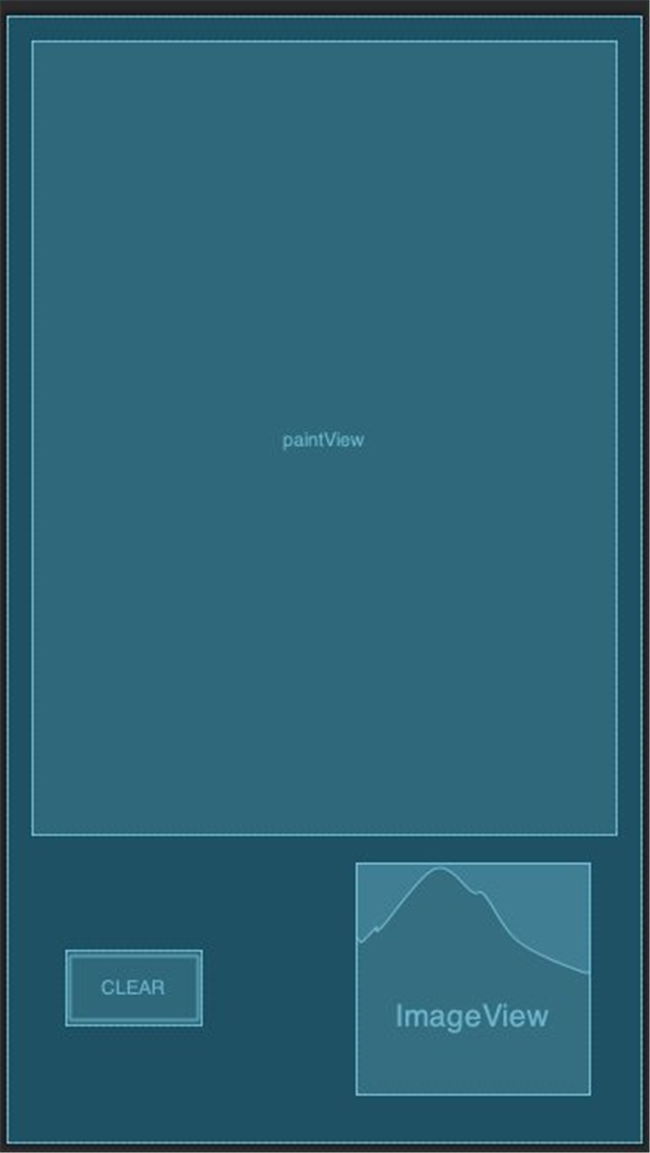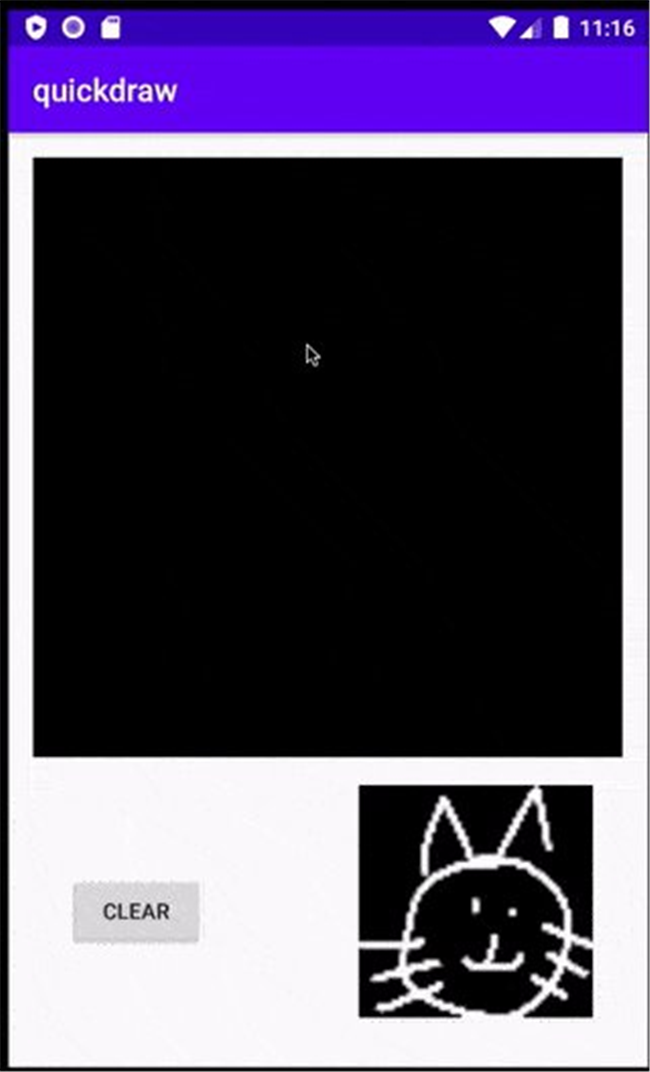## 第二部: 应对绘画动作

• touchStart: 感应触碰时触发
• touchMove: 当用户在屏幕上移动手指时触发
• touchUp: 当用户抬起手指时触发

### 重写 OnTouchEvent 和 OnDraw 方法

``````@Override
public boolean onTouchEvent(MotionEvent event) {
float x = event.getX();
float y = event.getY();

switch (event.getAction()) {
case MotionEvent.ACTION_DOWN :
touchStart(x, y);
invalidate();
break;
case MotionEvent.ACTION_MOVE :
touchMove(x, y);
invalidate();
break;
case MotionEvent.ACTION_UP :
touchUp();
runInference();
invalidate();
break;
}

return true;
}``````

``````@Override
protected void onDraw(Canvas canvas) {
canvas.save();
this.canvas.drawColor(DEFAULT_BG_COLOR);

for (Path path : paths) {
paint.setColor(DEFAULT_PAINT_COLOR);
paint.setStrokeWidth(BRUSH_SIZE);
this.canvas.drawPath(path, paint);
}
canvas.drawBitmap(bitmap, 0, 0, bitmapPaint);
canvas.restore();
}``````

### touchStart

``````private void touchStart(float x, float y) {
path = new Path();
path.reset();
path.moveTo(x, y);
this.x = x;
this.y = y;
}``````

### touchMove

``````private void touchMove(float x, float y) {
if (x < 0 || x > getWidth() || y < 0 || y > getHeight()) {
return;
}
float dx = Math.abs(x - this.x);
float dy = Math.abs(y - this.y);

if (dx >= TOUCH_TOLERANCE || dy >= TOUCH_TOLERANCE) {
path.quadTo(this.x, this.y, (x + this.x) / 2, (y + this.y) / 2);
this.x = x;
this.y = y;
}
}``````

### touchUp

``````private void touchUp() {
path.lineTo(this.x, this.y);
}``````

## Step 3: 开始推理

• 从URL读取模型
• 构建前处理和后处理过程
• 从 PaintView 进行推理任务

### 读取模型

DJL内建了一套模型管理系统。开发者可以自定义储存模型的文件夹。

``````File dir = getFilesDir();
System.setProperty("DJL_CACHE_DIR", dir.getAbsolutePath());``````

• `doodle_mobilenet.pt`: PyTorch模型
• `synset.txt`: 内包含分类任务中所有类别的名称
``````Criteria<Image, Classifications> criteria =
Criteria.builder()
.setTypes(Image.class, Classifications.class)
.optModelUrls("https://djl-ai.s3.amazonaws.com/resources/demo/pytorch/doodle_mobilenet.zip")
.optTranslator(translator)
.build();

``````@Override
protected Boolean doInBackground(Void... params) {
try {
predictor = model.newPredictor();
return true;
} catch (IOException | ModelException e) {
Log.e("DoodleDraw", null, e);
}
return false;
}``````

### 用Translator定义前处理和后处理

``````ImageClassificationTranslator.builder()
.optFlag(Image.Flag.GRAYSCALE)
.optApplySoftmax(true).build());``````

### Run inference from PaintView

``````public void runInference() {
// 拷贝图像
Bitmap bmp = Bitmap.createBitmap(bitmap);
// 缩放图像
bmp = Bitmap.createScaledBitmap(bmp, 64, 64, true);
// 执行推理任务
Classifications classifications = model.predict(bmp);
// 展示输入的图像
Bitmap present = Bitmap.createScaledBitmap(bmp, imageView.getWidth(), imageView.getHeight(), true);
imageView.setImageBitmap(present);
// 展示输出的图像
if (messageToast != null) {
messageToast.cancel();
}
messageToast = Toast.makeText(getContext(), classifications.toString(), Toast.LENGTH_SHORT);
messageToast.show();
}``````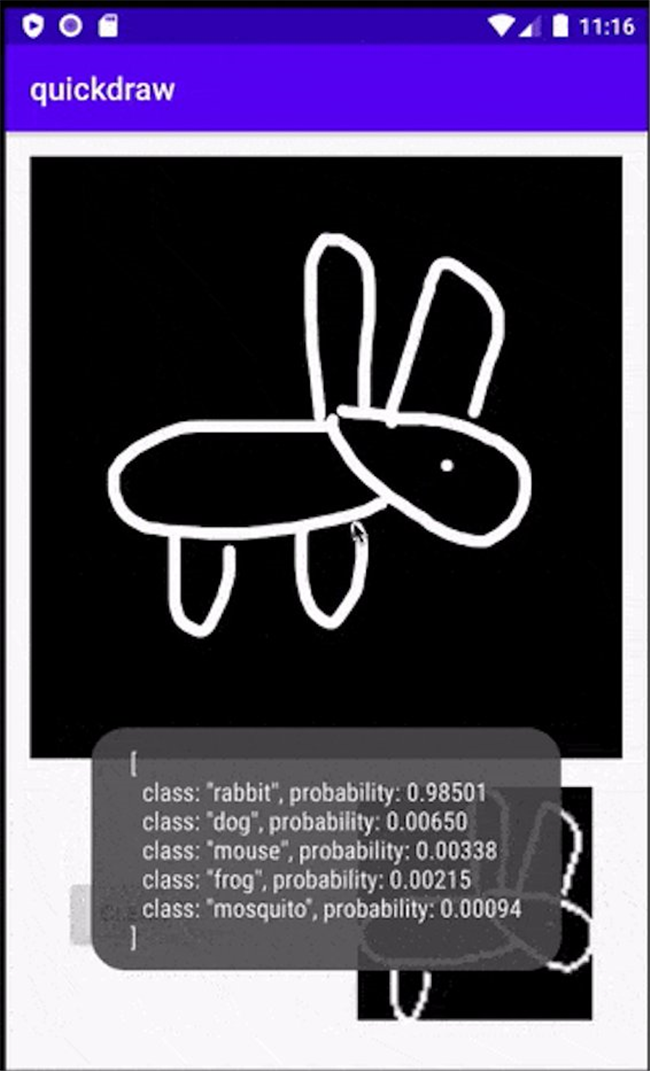## Optional: Optimize input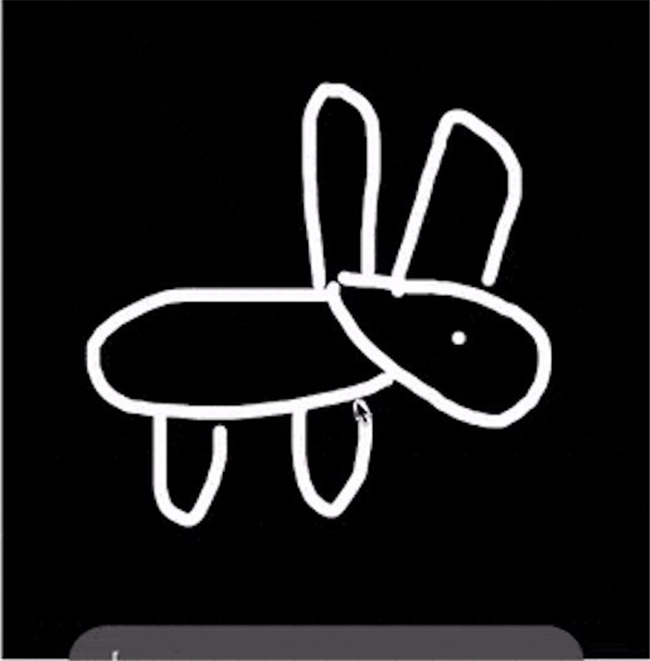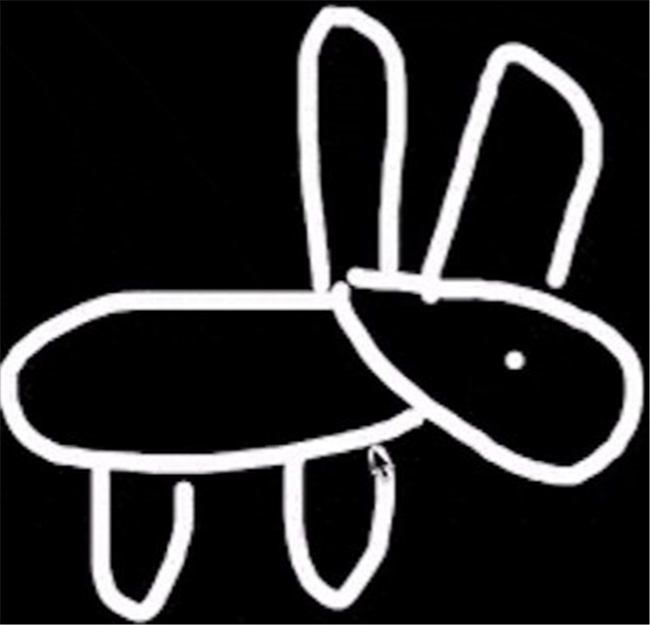``````RectF bound = maxBound.getBound();
int x = (int) bound.left;
int y = (int) bound.top;
int width = (int) Math.ceil(bound.width());
int height = (int) Math.ceil(bound.height());
// 截取部分图像
Bitmap bmp = Bitmap.createBitmap(bitmap, x, y, width, height);``````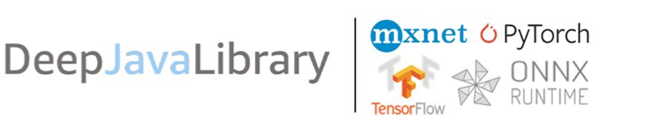Deep Java Library (DJL) 是一个基于 Java 的深度学习框架，同时支持训练以及推理。 DJL 博取众长，构建在多个深度学习框架之上 (TenserFlow、PyTorch、MXNet 等) 也同时具备多个框架的优良特性。你可以轻松使用 DJL 来进行训练然后部署你的模型。

## 本篇作者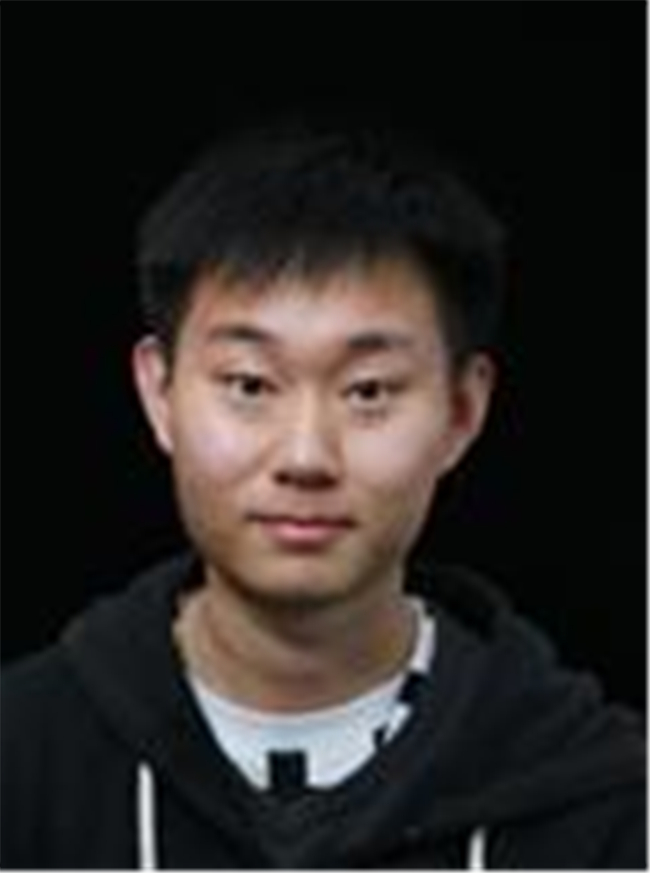### 兰青

Qing Lan, AWS AI 软件开发工程师。DJL深度学习框架作者之一，Apache软件基金会项目管理委员会成员。

TAGS: